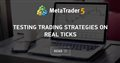# Opening position on sharp price movement33

Hi all,

Can someone help me with the below please?

I have developed this piece of code, which aims to open a position following a sharp variation in price in a given time-span.
If the price rises by at least 550 points in 2 candles, then the robot opens a SELL position.
If the price drops by at last 550 points in 2 candles, then the robot opens a BUY position.

Furthermore, the code is written to open a position only outside of the candles, and only if there are no other positions open.

I have 2 problems with this code.

1. The first and major problem is that the robot doesn't always capture the price variation. Meaning, that even it the price has moved by the minimum threshold, the robot doesn't open a position. It is visible even when backtesting. I recommend checking with gold or Brent. Both are suitable for the inputs already in the code.

2. The second problem is that the code works only with a minimum of 2 candles. If CloseB is set to be 1 candle behind rather than 2, the robot makes 0 trades.

CODE

```#property strict
//+------------------------------------------------------------------+
//INPUTS
input double Lots =0.1;
input double Threshold =550;
input double TP =600;
input double SL =800;

//+------------------------------------------------------------------+
void OnTick(void)
{
//+------------------------------------------------------------------+
//DO NOT TRADE ON OPEN BARS ONLY
if (Time >= TimeCurrent())
{
//+------------------------------------------------------------------+
//BEGINNING
if(OrdersTotal()<1)
{
double CloseA=iClose(0,0,0);
double CloseB=iClose(0,0,2);
//SELL IF PRICES RISES BY THRESHOLD
if((CloseA-CloseB)>=(Point*Threshold))
{
int ticket=OrderSend(Symbol(),OP_SELL,Lots,Bid,0,Bid+(SL*Point),Bid-(TP*Point),NULL,0,0,Red);
return;
}
//BUY IF PRICES LOWERS BY THRESHOLD
if((CloseB-CloseA)>=(Point*Threshold))
{
return;
}
}}}
```